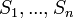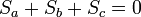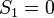# AKT-09/Tricolourability

Define the variables$S_1 , ... , S_n$ which are associated with the arcs of a knot diagram D. Each crossing yields an equation of the form$S_a + S_b + S_c = 0$. We can also (without loss of generality) assume$S_1 = 0$. Let M be the matrix over Z/3Z encoding the aforementioned relations. The nullity of M is non-zero if and only if there is a valid tricolouring of D.